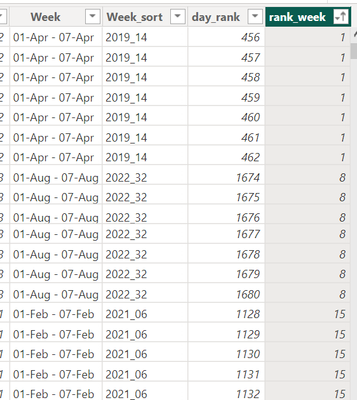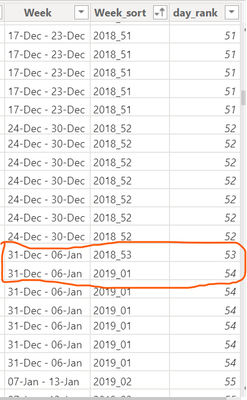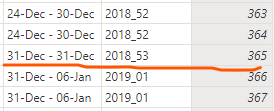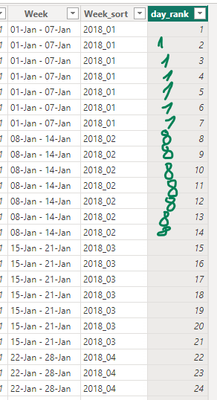cancel
Showing results for
Did you mean:Helper I

## Week sort

Hi all!

I have a column Week , which contains begging and end of week,

I was planning to sort it by date, but power bi returns an error "Sort by another column" ,

stating that there are multiple values in Column Date for Column Week, which I was trying to sort,

Therefore , I created column Week_sort that technically should be working, but it doesn't coz at the end of the year a row from column Week links to two different values from column Week_sort ,any ideas how to solve this problem?

my initial idea was to:

day_rank =
RANKX(
'Calendar',
'Calendar'[Date],,ASC)

which returns ranking of days,

and then "somehow" make DAX to repeat value from row above if row values in column Week are equal?

unfortunatelly i couldn't figure it out on my ownp.s. this table is created in DAX so I can't fix the problem in PQ

1 ACCEPTED SOLUTIONSuper User

@Ali_Shakh
You are digging in the wrong location. When to stop repeating? That brings you back to the original problem of ranking using the correct granularity. This is an infinite loop and will lead to nowhere.

The issue is that your business logic wasn't clarified so I had to guess. You can achieve this using

``````day_rank =
RANKX (
'Calendar',
'Calendar'[Date] - WEEKDAY ( 'Calendar'[Date], 2 ),
ASC,
DENSE
)``````

12 REPLIES 12Impactful Individual

Hello @Ali_Shakh , if you are looking to only sort, then use the following added column

Week sort = FORMAT(Calendar[Date],"yyyyww")Helper I

hi @eliasayy ,

that's not gonna work 🙂Super User

Hi @Ali_Shakh
Try

``````day_rank =
RANKX ( 'Calendar', 'Calendar'[Week],, ASC, Dense )``````Helper I

hi @tamerj1 ,

sorry, I forgot to mention, that won't work coz Week is a text values, and ranking it doesn't give correct (compared to date) result, as you can see here, 14th week of April 2019 is ranked 1st , even though, there are weeks before itSuper User

@Ali_Shakh

``````day_rank =
RANKX (
'Calendar',
VALUE ( SUBSTITUTE ( 'Calendar'[Week_Sort], "_", "" ) ),
ASC,
Dense
)``````Helper I

same problem,

one value from Week column links to two values from Day_rank columnSuper User

@Ali_Shakh
These are in two different years. The week sort says one is the last week of 2018 and the other is the first week 2019.  You shuld fix the week sort column, either to consider these 7 days as the last week of 2018 or to consider then as the first week of 2019. I mean week and week sort must be alligned.Helper I

I see, but how? 🙂

Formula in Week column is:

Week =
FORMAT(
'Calendar'[Date] - WEEKDAY('Calendar'[Date],2)+1,
"dd-MMM")
&" - "&
FORMAT(
'Calendar'[Date] - WEEKDAY('Calendar'[Date],2)+7,
"dd-MMM")
and it's automatically catches those days, it there a way to limit which days fall into which week?Super User

@Ali_Shakh

``````Week =
FORMAT ( 'Calendar'[Date] - WEEKDAY ( 'Calendar'[Date], 2 ) + 1, "dd-MMM" ) & " - "
& FORMAT (
MIN (
'Calendar'[Date] - WEEKDAY ( 'Calendar'[Date], 2 ) + 7,
ENDOFYEAR ( 'Calendar'[Date] )
),
"dd-MMM"
)``````Helper I

your solution works @tamerj1  and will accept it,
but it creates this short week containing only one day, and I know my colleagues will ask why 😅do you maybe know if it's possible to somehow repeat a value from row above on condition from other column,
like here, I did rankx of Date column

day_rank =
RANKX(
'Calendar',
'Calendar'[Date],,ASC)
and maybe it's possible to write a logical function ( using REPT, IF, EARLIER) that will check if next row in Week column is equial to previous one, if true, then repeat Day_rank row, if not then Day_rank?Super User

@Ali_Shakh
You are digging in the wrong location. When to stop repeating? That brings you back to the original problem of ranking using the correct granularity. This is an infinite loop and will lead to nowhere.

The issue is that your business logic wasn't clarified so I had to guess. You can achieve this using

``````day_rank =
RANKX (
'Calendar',
'Calendar'[Date] - WEEKDAY ( 'Calendar'[Date], 2 ),
ASC,
DENSE
)``````Helper I

@tamerj1 ,
well the last solution actually works fine, thanks again 🙂